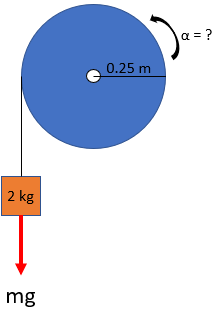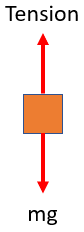## Angular Acceleration

Consider a 0.25 m radius wheel rotates about a frictionless axle in its center, and has a mass moment of inertia of 0.055 kg*m^2. If a 2 kg mass hangs off the wheel's side by a massless cord wrapped around the cylinder without slip, what is the wheel's angular acceleration?Hint
$$\sum M_{center}=I_{center}\times \alpha$$$where $$\alpha$$ is the angular acceleration both about an axis normal to the plane of motion, $$I$$ is the mass moment of inertia about the normal axis through the mass center, and $$M$$ is the moment. Hint 2 First, let's create the free body diagram and solve for the sum of forces in the y-direction:$$\sum F_y=mass \times acceleration$$$
First, let's create the free body diagram and solve for the sum of forces in the y-direction:$$\sum F_y=mass \times acceleration$$$$$mg-T=ma$$$
Because there is no slip: $$a=\alpha r$$
$$mg-T=m\alpha r$$$$$(2kg)(9.8m/s^2)-T=(2kg)\alpha (0.25m)$$$
$$T=(19.6kg\cdot m/s^2)-\alpha (0.5kg\cdot m)$$$In general, Newton's second law for a rigid body, with constant mass and mass moment of inertia, in plane motion may be written in vector form as $$\sum M_{center}=I_{center}\times \alpha$$$
where $$\alpha$$ is the angular acceleration both about an axis normal to the plane of motion, $$I$$ is the mass moment of inertia about the normal axis through the mass center, and $$M$$ is the moment. Since $$M=force \times distance$$ :
$$T(0.25m)=(0.055kg\cdot m^2)(\alpha)$$$$$[(19.6kg\cdot m/s^2)-\alpha (0.5kg\cdot m)](0.25m)=(0.055kg\cdot m^2)(\alpha)$$$
$$(4.9kg\cdot m^2\cdot s^{-2})-\alpha (0.125kg\cdot m^2)=(0.055kg\cdot m^2)(\alpha)$$$$$(4.9s^{-2})=(0.18)\alpha$$$
$$\alpha=\frac{4.9s^{-2}}{0.18}=27.2\:s^{-2}$$$$$27.2\:s^{-2}$$$